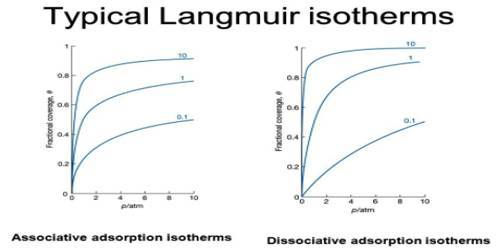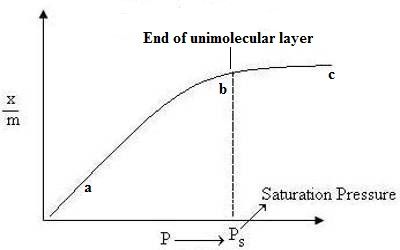Chemistry

# Langmuir Isotherm for Solid-liquid AdsorptionClassical isotherms of adsorption (Frumkin, Langmuir, Henry) were based on the model of nonpenetrable interface, where an adsorbate can substitute only molecules of one solvent.

Langmuir’s theory of unimolecular layer can be extended in an exactly analogous manner to adsorption from solutions. The workable form is at once obtained by putting C in place of and x/m in place of V, a quite justifiable procedure. The linear equation (Gas-Solid Systems: Langmuir Isotherm) then becomes

[C / (x/m)] = C/k1 + 1/k2

so that a plot of [C / (x/m)] vs C gives a straight line (k1 and k2 being constants).

Just as the equation is obeyed by many systems so also there are many cases which deviate from it. The deviation principally arises due to multilayer formation. In Figure, a linear plot of [C/(x/m)] vs C is shown for adsorption of jute iodine in CC14. Along ab Langmuir equation is obeyed; at ‘b’ the available surface is fully covered with a monolayer of iodine and between be, multilayer is formed. The general pattern of Figure is identical to the pattern as in Figure. It is possible to calculate the surface area of jute or any such adsorbent by using Langmuir isotherm. The necessary data are obtained from the slope and intercept of the linear portion ab.Figure: Adsorption of I2 on jute in CCl4 medium (schematic)